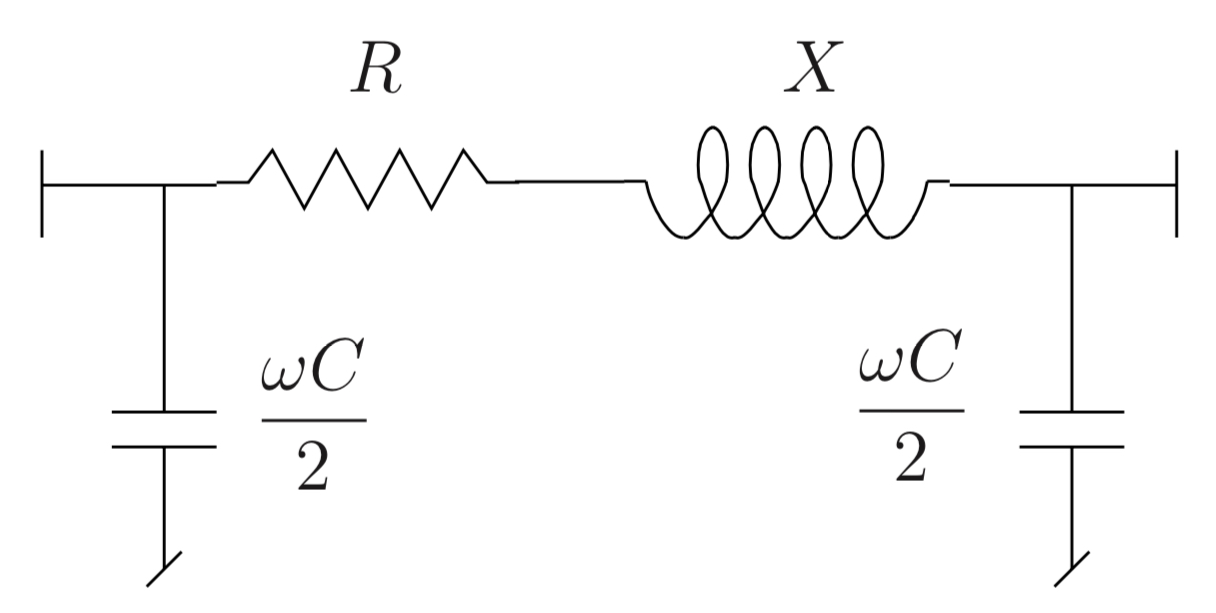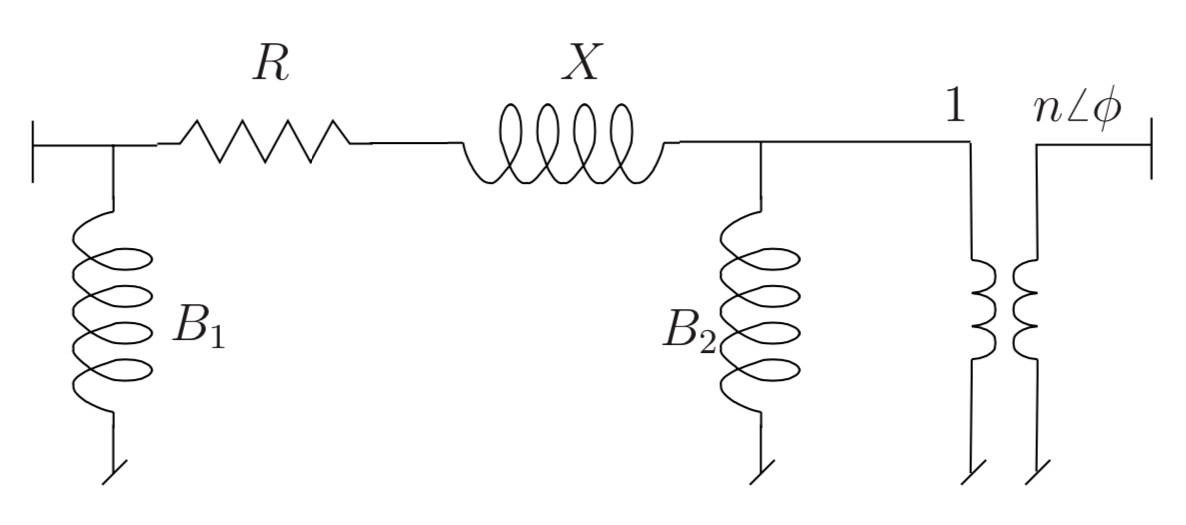# 1.1. Static data

The static data describe the topology of the network and an initial power flow solution from which the dynamic models are initialised. The static data are the parameters related to the buses, lines, and transformers of the system. The initial operating point is defined by the voltage magnitude and phase on each node.

## 1.1.1. Data used by RAMSES

BUS name vnom pload qload bshunt qshunt ;

Defines a bus in the network.

Parameters
• name (str) – (max 8 characters) name of the bus

• vnom (float) – base voltage, in kV. VNOM is used to set in per unit the parameters of the lines and transformers incident to the bus

• pload (float) – active power load, en MW

• qload (float) – reactive power load, en Mvar

• bshunt (float) – nominal reactive power, in Mvar, of the shunt compensation treated as constant admittance. This is the reactive power produced under a 1 pu voltage. A positive (resp. negative) value corresponds to a capacitor (resp. an inductor)

• qshunt (float) – nominal reactive power, in Mvar, of the shunt compensation treated as constant power in load flow computation. A positive (resp. negative) value corresponds to a capacitor (resp. an inductor).

Note

Only the name and vnom are considered in RAMSES. The other fields are ignored.

LINE name from to R X WC2 SNOM BR ;

The LINE record describes a line, a cable or a series capacitor.Parameters
• name (str) – (max 20 characters) name of the line

• from (str) – (max 8 characters) name of the “from” bus (the line orientation is arbitrary)

• to (str) – (max 8 characters) name of the “to” bus

• R (float) – resistance R, in $$\Omega$$

• X (float) – reactance X, in $$\Omega$$

• WC2 (float) – half shunt susceptance $$\omega C/2$$, in μS

• SNOM (float) – nominal apparent power, in MVA. This value should be set to zero if one does not want to specify this power; this will be interpreted as an infinite power.

• BR (int) – on/off status of the line breakers. A zero value indicates that the breakers are open at both ends; any other value means that both breakers are closed.

TRANSFO name from to R X B1 B2 N PHI SNOM BR ;

Transformer record.Parameters
• name (str) – (max 20 characters) name of transformer

• from (str) – (max 8 characters) name of the bus on the “1” side of the ideal transformer

• to (str) – (max 8 characters) name of the bus on the “n” side of the ideal transformer

• R (float) – resistance R, in % on the (VB1, SNOM) base

• X (float) – reactance X, in % on the (VB1, SNOM) base

• B1 (float) – susceptance B1, in % on the (VB1, SNOM) base. This is normally a negative value. It can be set to zero to account for the fact that in some phase-shifting transformers, n varies with the phase angle shift φ

• B2 (float) – susceptance B2, in % on the (VB1, SNOM) base. This is normally a negative value. It can be set to zero

• N (float) – ratio n, in % on the (VB1,VB2) base

• phi (float) – phase angle φ, in degrees

• SNOM (float) – apparent nominal power of the transformer, in MVA. This value must not be zero

• BR (int) – on/off status of the line breakers. A zero value indicates that the breakers are open at both ends; any other value means that both breakers are closed.

TRFO name from to con R X B N SNOM NFIRST NLAST NBPOS TOLV VDES BR ;

The TRFO record is a simplified variant of the TRANSFO and LTC-V records combined. n vary linearly with the tap position while X is constant. B2 and $$\phi$$ are zero.

Parameters
• name (str) – (max 20 characters) name of transformer

• from (str) – (max 8 characters) name of the bus on the “1” side of the ideal transformer

• to (str) – (max 8 characters) name of the bus on the “n” side of the ideal transformer

• con (str) – name of the bus whose voltage is controlled by adjusting the transformer ratio n. This must be one of the two terminal buses of the transformer, otherwise the program stops. An empty or blank string of characters is used to indicate that no voltage is controlled, i.e. the transformer ratio is fixed

• R (float) – resistance R, in % on the (VB1, SNOM) base

• X (float) – reactance X, in % on the (VB1, SNOM) base

• B (float) – susceptance B, in % on the (VB1, SNOM) base. This is normally a negative value. It can be set to zero

• N (float) – ratio n, in % on the (VB1,VB2) base

• SNOM (float) – apparent nominal power of the transformer, in MVA. This value must not be zero

• NFIRST (float) – ratio n, in %, corresponding to the first tap position

• NLAST (float) – ratio n, in %, corresponding to the last tap position

• NBPOS (float) – the number of tap positions

• TOLV (float) – tolerance $$\epsilon$$ (or half deadband) of the voltage control, in per unit

• VDES (float) – the setpoint voltage at the controlled bus, in per unit. As long as the controlled voltage differs from VDES by less than TOLV, the tap position remains unchanged

• BR (int) – on/off status of the line breakers. A zero value indicates that the breakers are open at both ends; any other value means that both breakers are closed.

Note

The parameters NFIRST NLAST NBPOS TOLV VDE are ignored in RAMSES. Any LTC type behaviour should be implemented in the dynamic data as a discrete controller.

LFRESV name mag phase ;

A LFRESV record specifies the voltage magnitude and phase angle at a bus.

Parameters
• name (str) – (max 8 characters) name of the bus

• mag (float) – voltage magnitude, in per unit

• phase (float) – voltage phase angle, in radian.

## 1.1.2. Data used only for the Power Flow and ignored by RAMSES

GENER name bus mon p q vimp snom qmin qmax br ;

In load flow computation, generators are described by GENER records, as follows.

Parameters
• name (str) – (max 10 characters) name of the generator

• bus (str) – (max 8 characters) name of the bus to which the generator is connected

• mon (str) – (max 8 characters) not used by ARTERE. To be set to CON BUS

• p (str) – active power production, in MW

• q (str) – reactive power production, in Mvar

• vimp (str) – imposed voltage, in per unit. If VIMP is zero, the generator is treated as a PQ bus with the reactive power production set at the Q value. Otherwise, it is treated as a PV bus and the Q field is ignored

• snom (str) – nominal apparent power, in MVA. This parameter must not be zero if one is willing to model the generator in detail (i.e. through a GROUP3 record) in the dynamic simulation

• qmin (str) – lower reactive power limit, in Mvar

• qmax (str) – upper reactive power limit, in Mvar

• br (str) – on/off status of the generator breaker. A zero value indicates that the breaker is open, any other value means that it is closed.